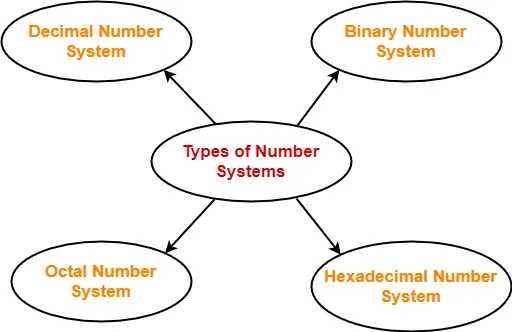## Base of Number System-

• Base of a number system is the total number of digits used in that number system.
• Number system with base ‘b’ has its digits in the range [0 , b-1].
• It is also called as radix of a number system.

## Examples-

Consider the following examples-

### Base 10 Number System-

Consider base 10 number system popularly called as decimal number system-

• Total number of digits used in this number system are 10 since it has base 10.
• These digits are 0 , 1 , 2 , 3 , 4 , 5 , 6 , 7 , 8 , 9.
• These digits clearly lie in the range [0 , base-1] = [0 , 9].

### Base 2 Number System-

Consider base 2 number system popularly called as binary number system-

• Total number of digits used in this number system are 2 since it has base 2.
• These digits are 0 and 1.
• These digits clearly lie in the range [0 , base-1] = [0 , 1].

## Important Note-

It is important to note that-

• All the digits of any number system with base ‘b’ are always less than ‘b’.
• This is clear from the range [0 , base-1] in which the digits of any number system lie.

## Types of Number System-

Four most commonly used number systems are-1. Decimal Number System
2. Binary Number System
3. Octal Number System

The following table shows the base and digits used in these number systems-

 Number System Base Digits Used Decimal Number System 10 0, 1, 2, 3, 4, 5, 6, 7, 8, 9 (Total 10 digits) Binary Number System 2 0, 1 (Total 2 digits) Octal Number System 8 0, 1, 2, 3, 4, 5, 6, 7 (Total 8 digits) Hexadecimal Number System 16 0, 1, 2, 3, 4, 5, 6, 7, 8, 9, A, B, C, D, E, F (Total 16 digits)

To gain better understanding about Basics of Number System,

Watch this Video Lecture

Next Article- Converting Any Base to Base 10

Get more notes and other study material of Number System.

Watch video lectures by visiting our YouTube channel LearnVidFun.